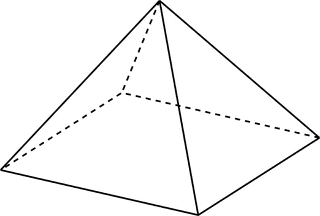# Geometry

## Objective

Identify and describe two-dimensional figures that result from slicing three-dimensional figures.

## Common Core Standards

### Core Standards

?

• 7.G.A.3 — Describe the two-dimensional figures that result from slicing three-dimensional figures, as in plane sections of right rectangular prisms and right rectangular pyramids.

?

• 5.G.B.3

• 5.MD.C.3

## Criteria for Success

?

1. Understand that a three-dimensional figure can be sliced in various ways that result in a two-dimensional cross-section
2. Understand that a slice through a right pyramid or prism, parallel to the base, will have the same shape as the base.
3. Identify the two-dimensional figures that result from various slices through right pyramids and prisms.

## Tips for Teachers

?

Visualizing the two-dimensional figures that result from slicing a three-dimensional figure can be challenging for some students. The following are two visual supports:

#### Remote Learning Guidance

If you need to adapt or shorten this lesson for remote learning, we suggest prioritizing Anchor Problem 2 (benefits from worked example). Find more guidance on adapting our math curriculum for remote learning here.

#### Fishtank Plus

• Problem Set
• Student Handout Editor
• Vocabulary Package

## Anchor Problems

?

### Problem 1

Justine has a block of cheese in the shape of a square prism, as shown below.She cuts the block in half.
What are two different two-dimensional faces that could result from Justine slicing the cheese in half?

### Problem 2

A right rectangular pyramid is shown below.Describe how the following two-dimensional figures can be created by slicing the pyramid.

• rectangle
• triangle
• trapezoid

## Problem Set

?The following resources include problems and activities aligned to the objective of the lesson that can be used to create your own problem set.